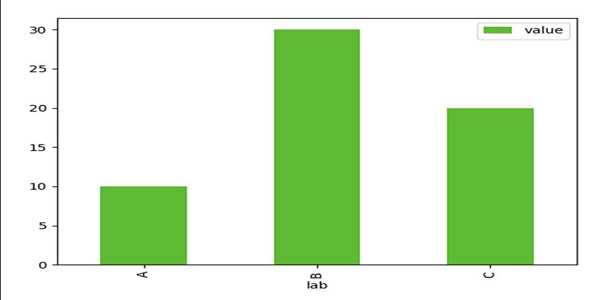# Annotate bars with values on Pandas bar plots in Python

In this program, we can create a data frame and can plot a bar using df.plot.bar(x='lab', y='value', color='#5fba34') plot.

## Steps

• Using Panda’s dataframe, we can create a data frame with the given dictionary, where the keys are lab and value, values of these keys are lists, respectively.

• Using Pandas plot.bar() method, we can create a vertical bar plot. A bar plot is a plot that presents categorical data with rectangular bars with lengths proportional to the values that they represent.

• To show the figure, use the plt.show() method.

## Example

import pandas as pd

from matplotlib import pyplot as plt
df = pd.DataFrame({'lab': ['A', 'B', 'C'], 'value': [10, 30, 20]})
ax = df.plot.bar(x='lab', y='value', color='#5fba34')
plt.show()

## Output